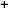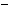Labware - MA35 Multivariable Calculus - Polar Coordinate Labs
 MA35 Labs » Polar Coordinate Labs Contents.1 Functions of Two Variables .1.1 Introduction .1.3 Slice Curves .1.4 Level Sets & Contour Lines .1.5 Continuity Search

Level Sets & Contour Lines

Text

The collection of all points [r,θ] in the domain of a function f for which f[r,θ] = c is called the level set of f at level c.Demos

 Contour LinesThis demo shows some level sets for the function f(r,t)= r2cos(2t). The level sets consist of level curves f(r,t) = c, and you can view them on the function graph in the "Function Graph: f(r,t)" window. In the "Domain" window, we show the level curves projected down into the rt-plane without actually displaying the function graph. This is called a "contour map", and it will prove to be very useful to look at in analyzing function graphs of functions f depending on more than two variables.Exercises

• Analyze Crater Lake shifted by an earthquake, with function f[r,θ]= -r4 + 2r2 + mrcos(θ) for various values of m. For which m will the lake no longer hold water? Describe the critical levels, i.e. the level sets that contain critical points.